# 六、接缝雕刻

• 什么是内容感知
• 如何量化和识别图像中有趣的部分
• 如何使用动态规划进行图像内容分析
• 如何在保持高度不变的情况下增加和减小图像的宽度而又不使兴趣区域恶化
• 如何使对象从图像中消失

# 我们为什么要关心接缝雕刻？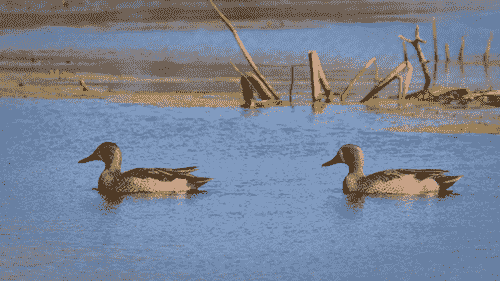# 它是如何工作的？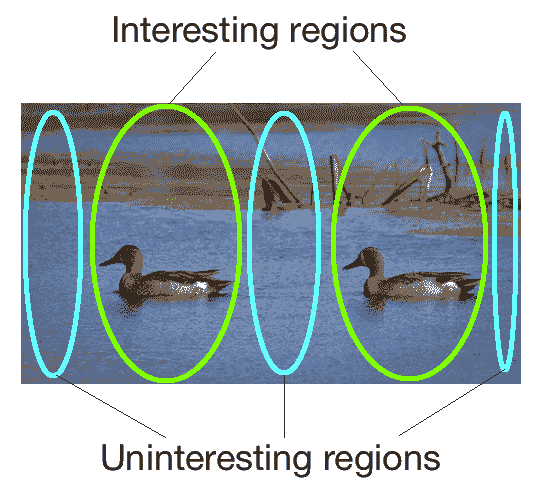# 我们如何计算接缝？

# Draw vertical seam on top of the image
def overlay_vertical_seam(img, seam):
img_seam_overlay = np.copy(img)

# Extract the list of points from the seam
x_coords, y_coords = np.transpose([(i,int(j)) for i,j in enumerate(seam)])

# Draw a green line on the image using the list of points
img_seam_overlay[x_coords, y_coords] = (0,255,0)
return img_seam_overlay

# Compute the energy matrix from the input image
def compute_energy_matrix(img):
gray = cv2.cvtColor(img, cv2.COLOR_BGR2GRAY)

# Compute X derivative of the image
sobel_x = cv2.Sobel(gray,cv2.CV_64F, 1, 0, ksize=3)

# Compute Y derivative of the image
sobel_y = cv2.Sobel(gray,cv2.CV_64F, 0, 1, ksize=3)

abs_sobel_x = cv2.convertScaleAbs(sobel_x)
abs_sobel_y = cv2.convertScaleAbs(sobel_y)

# Return weighted summation of the two images i.e. 0.5*X + 0.5*Y
return cv2.addWeighted(abs_sobel_x, 0.5, abs_sobel_y, 0.5, 0)

# Find vertical seam in the input image
def find_vertical_seam(img, energy):
rows, cols = img.shape[:2]

# Initialize the seam vector with 0 for each element
seam = np.zeros(img.shape)

# Initialize distance and edge matrices
dist_to = np.zeros(img.shape[:2]) + float('inf')
dist_to[0,:] = np.zeros(img.shape)
edge_to = np.zeros(img.shape[:2])

# Dynamic programming; iterate using double loop and compute the paths efficiently
for row in range(rows-1):
for col in range(cols):
if col != 0 and dist_to[row+1, col-1] > dist_to[row, col] + energy[row+1, col-1]:
dist_to[row+1, col-1] = dist_to[row, col] + energy[row+1, col-1]
edge_to[row+1, col-1] = 1

if dist_to[row+1, col] > dist_to[row, col] + energy[row+1, col]:
dist_to[row+1, col] = dist_to[row, col] + energy[row+1, col]
edge_to[row+1, col] = 0

if col != cols-1 and \
dist_to[row+1, col+1] > dist_to[row, col] + energy[row+1, col+1]:
dist_to[row+1, col+1] = dist_to[row, col] + energy[row+1, col+1]
edge_to[row+1, col+1] = -1

# Retracing the path
# Returns the indices of the minimum values along X axis.
seam[rows-1] = np.argmin(dist_to[rows-1, :])
for i in (x for x in reversed(range(rows)) if x > 0):
seam[i-1] = seam[i] + edge_to[i, int(seam[i])]

return seam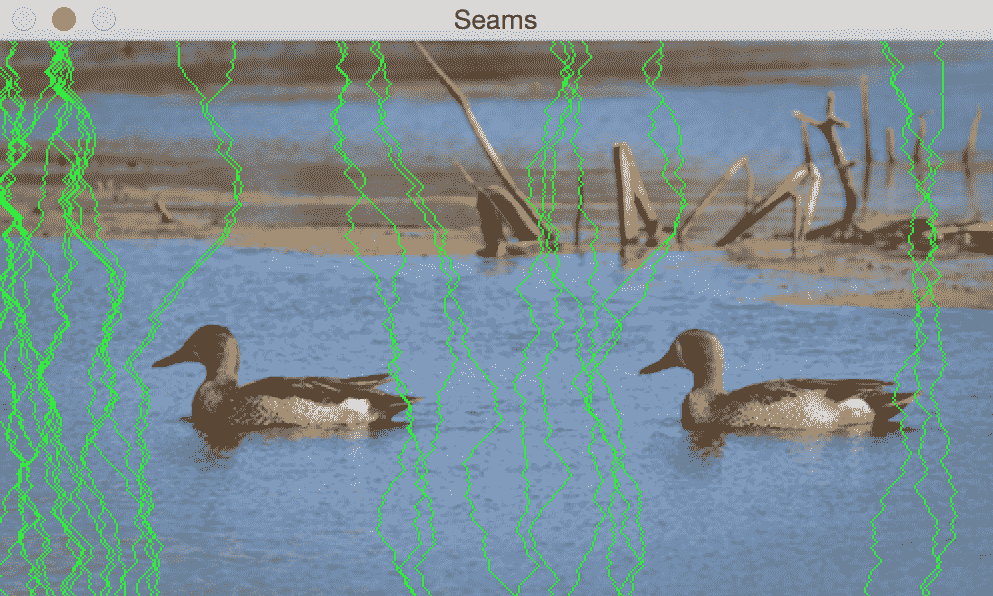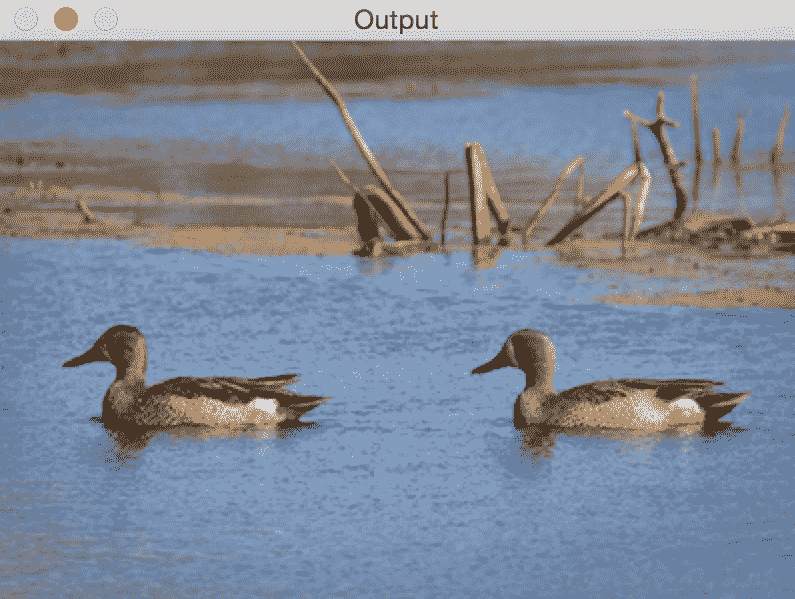import sys
import cv2
import numpy as np

# Remove the input vertical seam from the image
def remove_vertical_seam(img, seam):
rows, cols = img.shape[:2]

# To delete a point, move every point after it one step towards the left
for row in range(rows):
for col in range(int(seam[row]), cols-1):
img[row, col] = img[row, col+1]

# Discard the last column to create the final output image
img = img[:, 0:cols-1]
return img

if __name__=='__main__':
# Make sure the size of the input image is reasonable.
# Large images take a lot of time to be processed.
# Recommended size is 640x480\.

# Use a small number to get started. Once you get an
# idea of the processing time, you can use a bigger number.
# To get started, you can set it to 20\.
num_seams = int(sys.argv)

img = np.copy(img_input)
img_overlay_seam = np.copy(img_input)
energy = compute_energy_matrix(img)

for i in range(num_seams):
seam = find_vertical_seam(img, energy)
img_overlay_seam = overlay_vertical_seam(img_overlay_seam, seam)
img = remove_vertical_seam(img, seam)
energy = compute_energy_matrix(img)
print('Number of seams removed = ', i+1)

cv2.imshow('Input', img_input)
cv2.imshow('Seams', img_overlay_seam)
cv2.imshow('Output', img)
cv2.waitKey()



# 我们可以扩大图像吗？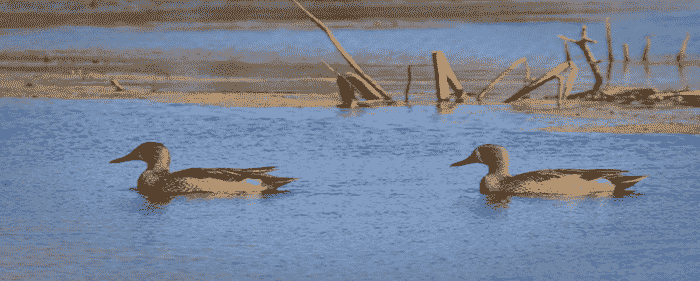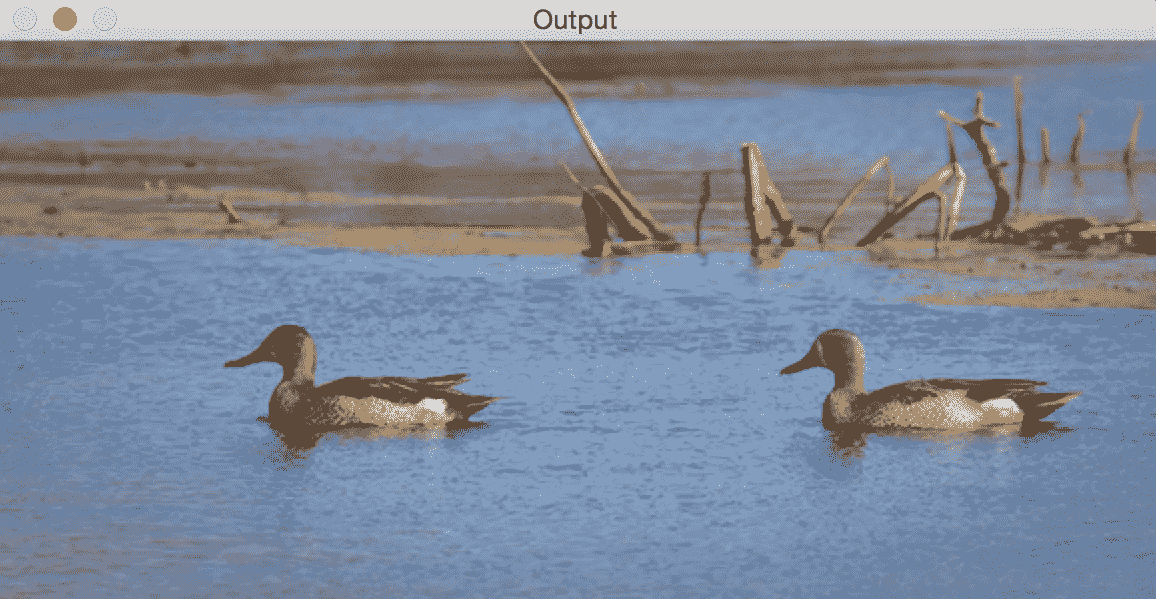import sys
import cv2
import numpy as np

# Add a vertical seam to the image
seam = seam + num_iter
rows, cols = img.shape[:2]
zero_col_mat = np.zeros((rows,1,3), dtype=np.uint8)
img_extended = np.hstack((img, zero_col_mat))

for row in range(rows):
for col in range(cols, int(seam[row]), -1):
img_extended[row, col] = img[row, col-1]

# To insert a value between two columns, take the average
# value of the neighbors. It looks smooth this way and we
# can avoid unwanted artifacts.
for i in range(3):
v1 = img_extended[row, int(seam[row])-1, i]
v2 = img_extended[row, int(seam[row])+1, i]
img_extended[row, int(seam[row]), i] = (int(v1)+int(v2))/2

return img_extended

if __name__=='__main__':
num_seams = int(sys.argv)

img = np.copy(img_input)
img_output = np.copy(img_input)
img_overlay_seam = np.copy(img_input)
energy = compute_energy_matrix(img) # Same than previous code sample

for i in range(num_seams):
seam = find_vertical_seam(img, energy) # Same than previous code sample
img_overlay_seam = overlay_vertical_seam(img_overlay_seam, seam)
img = remove_vertical_seam(img, seam) # Same than previous code sample
energy = compute_energy_matrix(img)
print('Number of seams added =', i+1)

cv2.imshow('Input', img_input)
cv2.imshow('Seams', img_overlay_seam)
cv2.imshow('Output', img_output)
cv2.waitKey()


# 我们可以完全去除对象吗？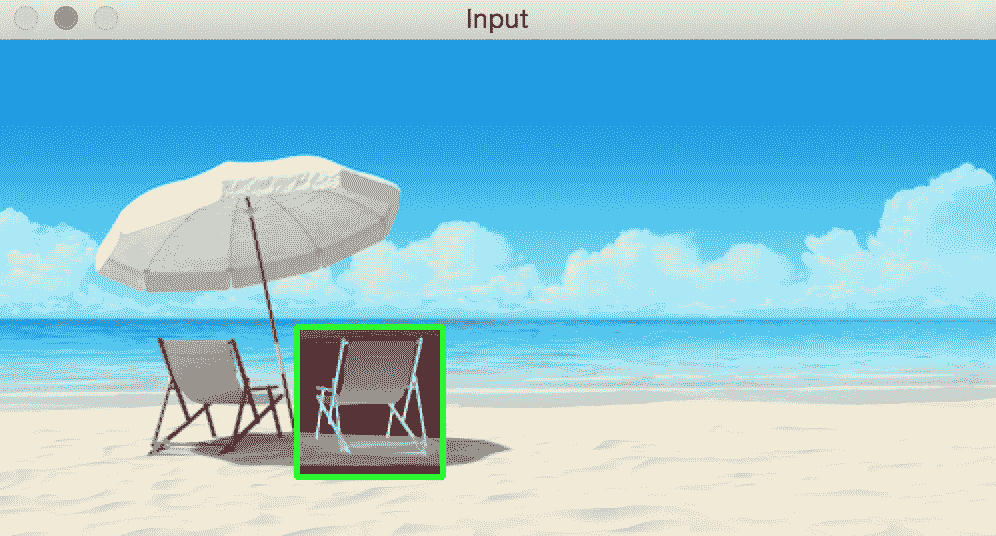import sys
import cv2
import numpy as np

# Draw rectangle on top of the input image
def draw_rectangle(event, x, y, flags, params):
global x_init, y_init, drawing, top_left_pt, bottom_right_pt, img_orig

# Detecting a mouse click
if event == cv2.EVENT_LBUTTONDOWN:
drawing = True
x_init, y_init = x, y

# Detecting mouse movement
elif event == cv2.EVENT_MOUSEMOVE:
if drawing:
top_left_pt, bottom_right_pt = (x_init,y_init), (x,y)
img[y_init:y, x_init:x] = 255 - img_orig[y_init:y, x_init:x]
cv2.rectangle(img, top_left_pt, bottom_right_pt, (0,255,0), 2)

# Detecting the mouse button up event
elif event == cv2.EVENT_LBUTTONUP:
drawing = False
top_left_pt, bottom_right_pt = (x_init,y_init), (x,y)

# Create the "negative" film effect for the selected
# region
img[y_init:y, x_init:x] = 255 - img[y_init:y, x_init:x]

# Draw rectangle around the selected region
cv2.rectangle(img, top_left_pt, bottom_right_pt, (0,255,0), 2)
rect_final = (x_init, y_init, x-x_init, y-y_init)

# Remove the object in the selected region
remove_object(img_orig, rect_final)

# Computing the energy matrix using modified algorithm
def compute_energy_matrix_modified(img, rect_roi):
# Compute weighted summation i.e. 0.5*X + 0.5*Y
energy_matrix = compute_energy_matrix(img)
x,y,w,h = rect_roi

# We want the seams to pass through this region, so make sure the energy values in this region are set to 0
energy_matrix[y:y+h, x:x+w] = 0

return energy_matrix

# Remove the object from the input region of interest
def remove_object(img, rect_roi):
num_seams = rect_roi + 10
energy = compute_energy_matrix_modified(img, rect_roi)

# Start a loop and rsemove one seam at a time
for i in range(num_seams):
# Find the vertical seam that can be removed
seam = find_vertical_seam(img, energy)

# Remove that vertical seam
img = remove_vertical_seam(img, seam)
x,y,w,h = rect_roi

# Compute energy matrix after removing the seam
energy = compute_energy_matrix_modified(img, (x,y,w-i,h))
print('Number of seams removed =', i+1)

img_output = np.copy(img)

# Fill up the region with surrounding values so that the size
# of the image remains unchanged
for i in range(num_seams):
seam = find_vertical_seam(img, energy)
img = remove_vertical_seam(img, seam)
energy = compute_energy_matrix(img)
print('Number of seams added =', i+1)

cv2.imshow('Input', img_input)
cv2.imshow('Output', img_output)
cv2.waitKey()

if __name__=='__main__':
drawing = False
img = np.copy(img_input)
img_orig = np.copy(img_input)

cv2.namedWindow('Input')
cv2.setMouseCallback('Input', draw_rectangle)
print('Draw a rectangle with the mouse over the object to be removed')
while True:
cv2.imshow('Input', img)
c = cv2.waitKey(10)
if c == 27:
break

cv2.destroyAllWindows()